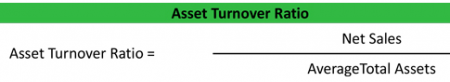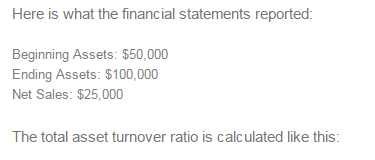# Asset Turnover Ratio: Mathematical Explanation

The asset turnover ratio is an efficiency ratio that measures a company’s ability to generate sales from its assets by comparing net sales with average total assets. In other words, this ratio shows how efficiently a company can use its assets to generate sales.

The total asset turnover ratio calculates net sales as a percentage of assets to show how many sales are generated from each dollar of company assets. For instance, a ratio of .5 means that each dollar of assets generates 50 cents of sales.

Formula:

The asset turnover ratio is calculated by dividing net sales by average total assets.Net sales, found on the income statement, are used to calculate this ratio returns and refunds must be backed out of total sales to measure the truly measure the firm’s assets’ ability to generate sales.

Average total assets are usually calculated by adding the beginning and ending total asset balances together and dividing by two. This is just a simple average based on a two-year balance sheet. A more in-depth, weighted average calculation can be used, but it is not necessary.

Analysis:

This ratio measures how efficiently a firm uses its assets to generate sales, so a higher ratio is always more favorable. Higher turnover ratios mean the company is using its assets more efficiently. Lower ratios mean that the company isn’t using its assets efficiently and most likely have management or production problems.

For instance, a ratio of 1 means that the net sales of a company equals the average total assets for the year. In other words, the company is generating 1 dollar of sales for every dollar invested in assets.

Like with most ratios, the asset turnover ratio is based on industry standards. Some industries use assets more efficiently than others. To get a true sense of how well a company’s assets are being used, it must be compared to other companies in its industry.

The total asset turnover ratio is a general efficiency ratio that measures how efficiently a company uses all of its assets. This gives investors and creditors an idea of how a company is managed and uses its assets to produce products and sales.

Example:

Sally’s Tech Company is a tech start up company that manufactures a new tablet computer. Sally is currently looking for new investors and has a meeting with an angel investor. The investor wants to know how well Sally uses her assets to produce sales, so he asks for her financial statements.As you can see, Sally’s ratio is only .33. This means that for every dollar in assets, Sally only generates 33 cents. In other words, Sally’s start up in not very efficient with its use of assets.